Mobile QR Code1. (Department of Electrical and Information Engineering, Seoul National University of Science and Technology, Seoul, Korea)

Analog front-end IC, neural recording amplifier, programmable gain amplifier, stimulation artifact

## I. INTRODUCTION

A neural signal recorder is used in implantable closed-loop neuromodulation systems to closely monitor and help diagnose neurological conditions [1,2]. The neural signals of interest occupy the 1 Hz to 5 kHz frequency band, which include local field potentials (LFP, 1-200 Hz) and action potentials (AP, 200 Hz-5 kHz), and are known to have small signal amplitudes of 1-mV or less .

In order to maintain the signal integrity over the processing chain, one of the key specification in the design of the neural recording analog front-end (AFE) is the integrated input-referred noise that must be kept less than the background noise over the desired bandwidth which is around 5 to 10 ${\mu}$Vrms [2-4]. Furthermore, power consumption must be kept minimal considering tissue heating in the surroundings and to place less burden on the wireless power delivery and management circuits. It is also desirable to have gain control capability in the AFE to handle various amplitudes of neural signals, as well as support tunable bandwidth to cater both LFP and AP simultaneously or separately. Considering multi-channel implementation of the AFE to interface with the multi-electrode array, it is important to minimize the circuit complexity and area, while satisfying the design specifications required by the application.

In addition to the above mentioned traditional design requirements of the recording AFE, stimulation artifacts generated by a concurrently operating stimulator must be considered in closed-loop neural interface systems [5-7]. As the stimulator usually drives the electrode with large amplitudes of voltage and current , the recording electrode and its interfacing circuit may suffer from large stimulation artifacts which couple into the input of the recorder, potentially saturating the recording path.

In this work, a simple AFE IC is proposed to provide good overall performance regarding input noise, power consumption, and artifact handling capability. The paper is organized as follows. Section II describes the overall architecture of AFE IC while Section III presents the circuit design details. Section IV discusses the experimental results followed by the conclusions in Section V.

## II. ARCHITECTURE AND DESIGN CONSIDERATIONS

Fig. 1 shows the simplified block diagram of the proposed AFE. The fully-differential AFE consists of a low-noise amplifier (LNA) and a programmable gain amplifier (PGA). In addition, a set of unity-gain buffers follow the PGA for testing purposes. Although chopper-stabilized amplifier topologies [5,7,9] are widely used for conditioning LFP signals and for less-invasive low-frequency neural recording applications such as in electrocorticography (ECoG) and electroencephalogram (EEG) to mitigate the flicker noise issue in the sub-200 Hz band, the overall complexity of the system increases significantly due to several auxiliary feedback loops with clock-driven switches to enable the operation and improve the performance of the chopper amplifier. On the other hand, as the proposed system targets wide bandwidth considering both LFPs and APs, this work opts for a simpler architecture without chopper stabilization. Thus, an AC-coupled input with capacitive-feedback architecture is employed for both the LNA and PGA, which is a simple, yet effective method to handle DC offsets generated from the electrode-electrolyte interface. To provide a power-efficient solution, it is crucial that the supply voltage and/or bias current must be minimized in the design while limiting the noise level. Considering the compromise between power consumption and the linearity of the AFE, a 1-V supply is used for all the design blocks.

Many of the previous neural AFE solutions allocate around 40 dB of closed-loop gain in the LNA stage considering the weak signal level and the noise performance of the recording system [2-4]. In this work, a moderate fixed closed-loop gain of 20 dB is allocated for the LNA considering the large artifacts which need to be accommodated along with the desired signal. The PGA stage provides additional programmable gain of 0 to 20 dB. In addition, the low-pass cutoff frequency of PGA can be set to 650 Hz or 6.5 kHz by controlling the bias current of its core operational transconductance amplifier (OTA). Thus for the acquisition of low-band neural signals such as LFP, ECoG, and EEG, the low-pass cutoff frequency can be set to 650 Hz. Likewise, for processing wide-band neural signals such as AP, it can be set to 6.5 kHz.

##### Fig. 1. Block diagram of proposed analog front-end IC.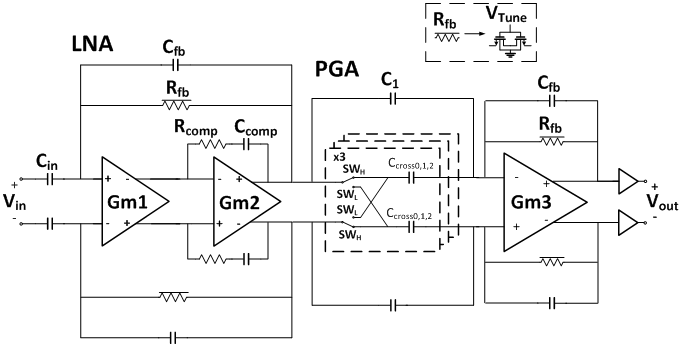## III. CIRCUIT DESCRIPTION

### 1. Neural Recording Amplifier

As shown in Fig. 1, the LNA utilizes an AC-coupled structure to reject the large DC offset generated by the electro-chemical reaction at the electrode-tissue interface. The mid-band closed-loop gain of LNA is determined by C$_{\mathrm{in}}$/C$_{\mathrm{fb}}$, which is the ratio of input capacitance C$_{\mathrm{in}}$, and feedback capacitance, C$_{\mathrm{fb}}$. The C$_{\mathrm{in}}$ and C$_{\mathrm{fb}}$ affect the noise performance of the LNA, and the equation of LNA noise can be calculated as:

##### (1)
$\overline{v_{in}^{2}}=\left(\frac{C_{in}+C_{fb}+C_{p}}{C_{in}}\right)^{2}\overline{v_{in\_ OTA}^{2}}$

where C$_{\mathrm{p}}$is the input parasitic capacitance of OTA and v$_{\mathrm{in,OTA}}$ is the input-referred noise of the OTA. In the proposed LNA, input capacitor and feedback capacitor is sized as 10 pF and 1 pF, respectively, considering the trade-off between its area and the noise performance. Thus, the mid-band gain of LNA is set to 20 dB. The high-pass cutoff frequency should be set to less than 1 Hz, which is determined by C$_{\mathrm{fb}}$ and the pseudo-resistor R$_{\mathrm{fb}}$ connected in parallel to the feedback capacitor. The gate control voltage of R$_{\mathrm{fb}}$, noted as V$_{\mathrm{Tune}}$, can also be used as a reset signal to quickly initialize the LNA. The low-pass cutoff frequency is determined by the transconductance of the OTA, closed-loop gain, and load capacitance.

The LNA utilizes two stages of OTA, noted as G$_{\mathrm{m1}}$ and G$_{\mathrm{m2}}$ in Fig. 1, to obtain large open-loop gain and wide output swing. Fig. 2 shows the circuit schematic of G$_{\mathrm{m1}}$, the first stage OTA. Among widely used topologies such as conventional two-stage OTA, current-mirror , complementary current-reused [3,5], inverter-stacked [4,11], a PMOS-input folded-cascode topology  is chosen for its large input range and relatively large open-loop gain characteristic. For enhanced power-noise efficiency, the OTA is modified for improved noise performance with trade-off in input range by utilizing the NMOS transistors M$_{3}$-M$_{4}$ as additional inputs for boosted transconductance. The input transistors M$_{1}$-M$_{4}$ are all biased in sub-threshold region for maximum g$_{\mathrm{m}}$/I$_{\mathrm{D}}$ ratio. To mitigate flicker noise, large size dimensions are employed for the input transistors with width/length ratio of 300 ${\mu}$m/1 ${\mu}$m and 200 ${\mu}$m/2.5 ${\mu}$m for PMOS and NMOS devices, respectively. The input-referred thermal noise of the proposed OTA circuit is as follows:

##### (2)
$\begin{equation} \begin{array}{l} \overline{v_{in,OTA}^{2}}\approx 8kT\gamma \left[\frac{1}{\left(g_{m1,2}+g_{m3,4}\right)}+\frac{g_{m7,8}}{\left(g_{m1,2}+g_{m3,4}\right)^{2}}\\ +\frac{g_{m9}}{\left(g_{m1,2}+g_{m3,4}\right)^{2}}\right] \end{array} \end{equation}$

where k is the Boltzman constant, T is the absolute temperature, ${\gamma}$ is excess noise coefficient, g$_{\mathrm{m1,2}}$and g$_{\mathrm{m3,4}}$ are the transconductance of PMOS and NMOS input transistors, respectively. Fig. 3 shows the second stage OTA of LNA which is a simple five-transistor fully-differential OTA for large output range. The first and second stage OTA, including the common-mode feedback (CMFB) circuits, consume 0.96 ${\mu}$W and 0.2~${\mu}$W of power, respectively, from 1 V supply. The simulated phase margin is 60$^{\circ}$ for both the main loop and CMFB loops.

##### Fig. 2. Circuit schematic of first stage OTA of LNA (G$_{\mathrm{m1}}$).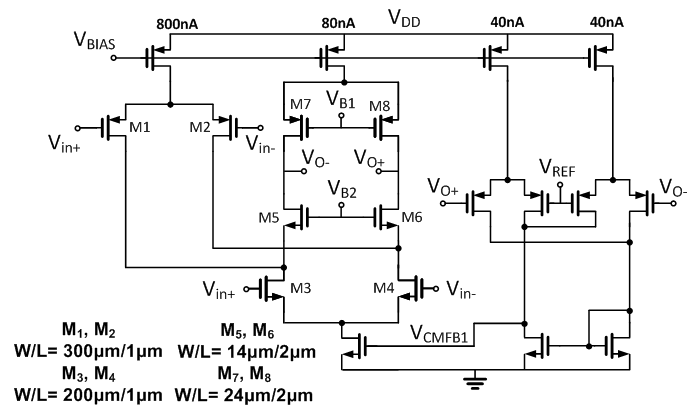##### Fig. 3. Circuit schematic of second stage OTA of LNA (G$_{\mathrm{m2}}$).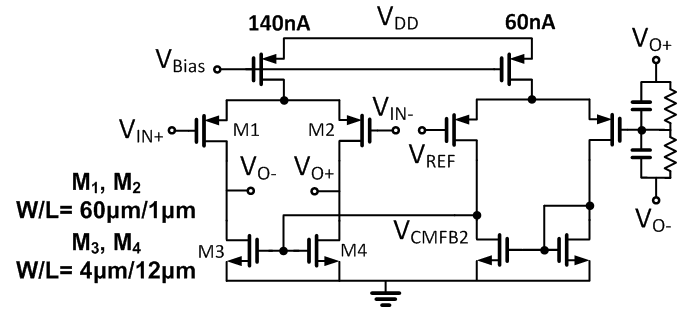### 2. Programmable Gain Amplifier (PGA)

The circuit schematic of the proposed PGA is shown in Fig. 4(a). The PGA follows the LNA and provides bandwidth control function and additional gain to the output of the LNA. The gain of the PGA is decided by the capacitor ratio C$_{\mathrm{in}}$/C$_{\mathrm{fb}}$, where C$_{\mathrm{in}}$ is controlled by a 3-bit switched cross-coupled capacitor structure shown in more detail in Fig. 4(b) to provide variable gain without distortion in the low-frequency range . The C$_{\mathrm{in}}$ is decided as follows:

##### (3)
$C_{in}=C_{1}+a_{1}C_{cross}$

When C$_{\mathrm{cross}}$ is connected with SW$_{\mathrm{H}}$, a parallel configuration results and a$_{1}$ has a value of +1. When connected with SW$_{\mathrm{L}}$, it results in a crossed configuration and a$_{1}$ has a value of -1. The PGA provides a variable gain from 0 dB to 20 dB, and the values of the capacitors used are shown in the table in Fig. 4(a).

The high-pass cutoff frequency f$_{\mathrm{HP}}$ of the PGA is determined by the feedback capacitor and the feedback pseudo-resistor of the PGA. The low-pass cutoff frequency f$_{\mathrm{LP}}$ of the PGA is set as follows:

##### (4)
$f_{LP}=\frac{G_{m}}{Gain\times C_{load}}$

where G$_{\mathrm{m}}$is the transconductance of the core OTA. Therefore, the low-pass cutoff frequency can be adjusted by controlling the transconductance of the PGA core amplifier. The circuit schematic core OTA is shown in Fig. 5, which employs a fully-differential current-mirror topology for wide output swing. The LP0 controls the tail current flowing through the core OTA, and as a result, the transconductance of the core OTA changes and the low-pass cutoff frequency can be controlled. The PGA is designed to be able to select a wide bandwidth of 1-6.5~kHz and a low frequency band of 1-650 Hz by controlling the current. When f$_{LP}$ = 650 Hz is set, the current flowing through the core OTA is 30 nA, and the current flowing through the CMFB is 10 nA. When f$_{LP}$=6.5-kHz is set, the current consumption of the core OTA is 240 nA, while the CMFB circuit consumes 90~nA.

##### Fig. 4. (a) Circuit schematic of PGA; (b) Cross-coupled capacitor.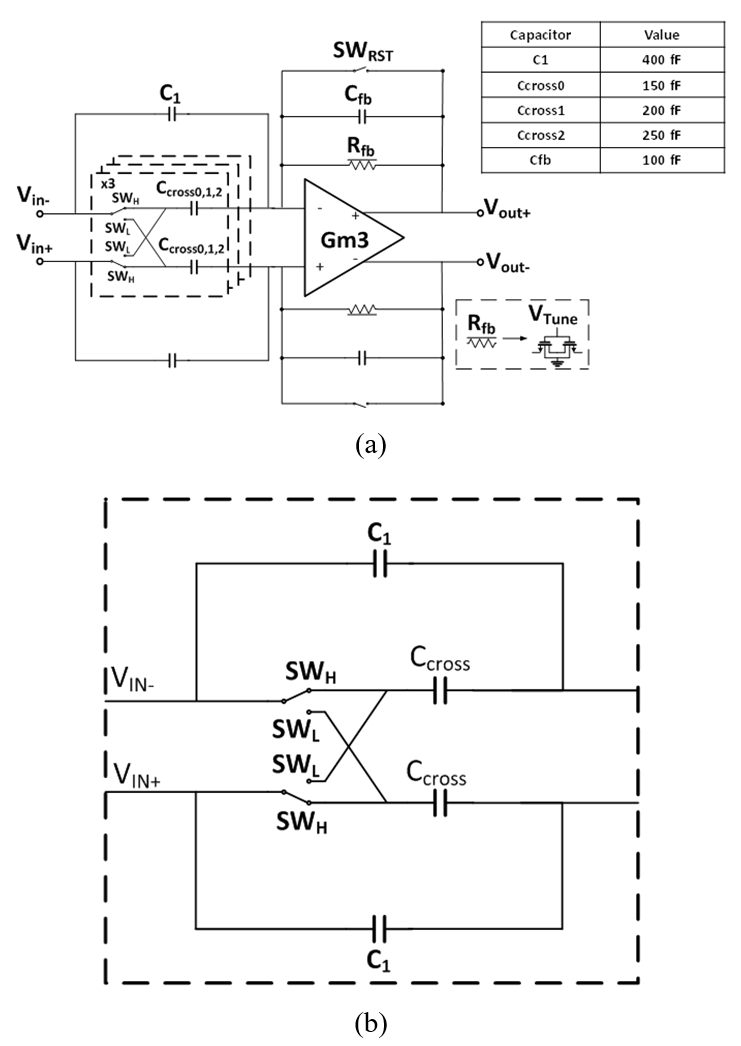##### Fig. 5. Circuit schematic of core OTA of PGA.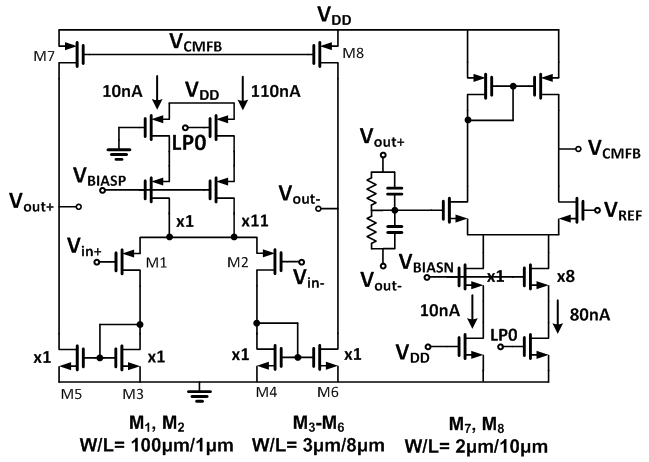## IV. MEASUREMENT RESULTS

Fig. 6 shows the chip micrograph of the proposed AFE IC. The chip was fabricated using 0.18 ${\mu}$m CMOS process, and occupies an area of 0.21 mm$^{2}$, which include the area of on-chip unity-gain buffers for testing purpose. The chip is packaged using chip-on-board method and a FR4 printed circuit board is used for testing. A 1-V supply voltage is applied to the IC through an on-board low dropout regulator. Excluding the power consumed in the measurement buffers, the AFE consumes 1.49 ${\mu}$W power in wide-band mode and 1.25~${\mu}$W power in low-band mode.

The measured ac response of AFE is shown in Fig. 7. The mid-band gain can be set from a minimum of 20 dB to a maximum of 40 dB, and the low-pass cutoff frequency f$_{\mathrm{LP}}$ can be controlled to 650 Hz or 6.5 kHz. Also, the high-pass cutoff frequency f$_{\mathrm{HP}}$was set at 300~mHz. Fig. 8 shows the input-referred noise plot of the AFE. The integrated input-referred noise is 1.98~${\mu}$V$_{\mathrm{rms}}$ in the low-band of 1-650 Hz and 3.39 ${\mu}$V$_{\mathrm{rms}}$ in the 1-6.5~kHz wide-band mode.

The noise efficiency factor (NEF) is a parameter measuring the power-to-noise performance of system , and expressed as follows:

##### (5)
$NEF=V_{in,rms}\sqrt{\frac{2I_{total}}{\pi \cdot V_{T}\cdot 4kT\cdot BW}}$

where V$_{\mathrm{in.rms}}$ is overall input-referred noise of AFE, I$_{\mathrm{total}}$ is the total current used by the AFE, V$_{\mathrm{T}}$ is the thermal voltage, and BW is the bandwidth of the AFE, respectively. The proposed AFE achieves NEF performance of 3.27 in the 1-650 Hz band and 1.93 in the 1-6.5 kHz band.

The performance regarding tolerance to CM artifacts is measured using a two-tone test. For testing, the frequency of the desired signal and CM artifact are assumed to be 1 kHz and 900 Hz, respectively. Fig. 9 shows the power spectrum of the AFE output when the desired input of 2 mV$_{\mathrm{pp}}$ is injected with the CM artifact of 280-mV$_{\mathrm{pp}}$. At each gain from 20 dB to 40 dB, the total harmonic distortion (THD) is 0.33%, 0.67%, 0.54%, and 0.66%. Fig. 10 presents the AFE output FFT response to 60 mVpp 1-kHz input signal at 20 dB gain which is the minimum gain setting of the AFE to handle large DM artifact. The third-order harmonic distortion is -46 dB and the THD is 0.88%. Therefore, for less than 1% of THD, the proposed AFE can be said to accommodate up to 280 mV$_{\mathrm{pp}}$ of CM artifact and 60 mVpp of DM artifact. The common-mode rejection ratio (CMRR) result is shown in Fig. 11, where it is measured to be greater than 72 dB over the band of interest (1 Hz - 6.5 kHz). Fig. 12 shows the pre-recorded action potential neural signal from an adult female Sprague Dawley rat of the open-source data set [14,15] which is injected to the input of the AFE through a waveform generator and Fig. 13 shows the measured transient output of AFE when the bandwidth is set at 6.5 kHz. According to the gain control, the magnitudes of the signals are amplified by 20, 26, 34, and 40 dB, respectively. Fig. 14 shows the power spectrum density of the output waveform according to the low-pass cutoff frequency control function of the PGA when the pre-recorded AP signal is injected to the AFE. In the low-band mode, f$_{\mathrm{LP}}$ is set to 650 Hz, and in the wide-band mode, f$_{\mathrm{LP}}$ is set to 6.5 kHz. Therefore, in both modes, the power spectrum has the almost same magnitude in the 1-650 Hz band, but in the low-band mode, the signals are filtered in the high frequency band.

The measured performance is summarized in Table 1 and compares with previous AFE ICs for neural recording applications. References [3,4,16] are all comprised of LNA and PGA and are fabricated using similar process technologies. Although these works show good overall results in key parameters, the work in  has sub-optimal noise performance while reference  has low input noise but its power consumption can be improved. Reference  has a good noise performance with small area, but its power consumption is quite high. All three works also do not mention or provide results regarding the artifact tolerance and thus may not be applicable for closed-loop neural interface applications. References [5,7] are chopper modulated LNAs with active artifact cancellation functions in the analog domain to handle large common-mode interference including stimulation artifacts and power line interference. Both works provide good measured results in handling large artifact with comparable overall performance. However, the design complexity is quite high despite just having a single LNA block with sub-optimal power efficiency and noise performance and limited flexibility in gain and bandwidth control. On the other hand, using a simple design approach, this work offers a viable power-efficient AFE solution with well-balanced overall performance suitable for closed-loop bidirectional neural system-on-chips (SoC).

##### Table 1. Performance summary of AFE IC
 Parameter      This work Blocks LNA+PGA LNA+PGA+BUF LNA LNA LNA+PGA LNA+PGA Power/ch 2.28 μW 2.82 μW 2.8 μW 2.27 μW 9 μW 1.49 μW Supply 1 V 1 V 1.2 V 0.8 V 1.8 1 V Gain 43-61 dB 45-63 dB 25.7 dB 43.3 dB 35-49.5 dB 20~40 dB BW 1 Hz~8.9 kHz 1 Hz~9 kHz 1 Hz~5 kHz 1 Hz~7.5 kHz 0.7 Hz~9.3 kHz 1~650 Hz (LB) 1 Hz~6.5 kHz (WB) CMRR 90.2 dB (sim.) >80 dB (sim.) 78 dB (@50 Hz) >100 dB(@50 Hz) 76 dB >70 dB (@1 kHz) IRN(Vrms) 4.74 μVrms (1 Hz~10 kHz) 3.16 μVrms (1 Hz~10 kHz) 1.8 μVrms (1~200 Hz) 5.3 μVrms (0.2 k~5 kHz) 1.2 μVrms (1~650 Hz) 4.1 μVrms (1 Hz~7.5 kHz) 3.2 μVrms (1 Hz~10 kHz) 1.98 μVrms (1~650 Hz) 3.39 μVrms (1 Hz~6.5 kHz) NEF 2.91 (1 Hz~10 kHz) 2.04 (1 Hz~10 kHz) 7.4(1~200 Hz) 4.4(0.2 k~5 kHz) 3.06(1~650 Hz) 3.08(1 Hz~7.5 kHz) 1.94 (LNA only, 1 Hz~10 kHz) 3.27(1~650 Hz) 1.93(1 Hz~6.5 kHz) PEF (NEF$^{2}$ VDD) 8.47 (1 Hz~10 kHz) 4.16 (1 Hz~10 kHz) 65.7(1~200 Hz) 23.3(0.2 k~5 kHz) 7.49(1~650 Hz) 7.59(1 Hz~7.5 kHz) 6.77. (LNA only, 1 Hz~10 kHz) 10.7 (1~650 Hz) 3.71 (1 Hz~6.5 kHz) Artifact Tolerance N/A N/A up to 650 mVpp up to 600 mVpp N/A up to 280 mVpp Max. input N/A N/A 80 mVpp 4 mVpp 24 mVpp (@gain=35 dB) 60 mVpp (@gain=20 dB) Chopping X X O O X X Area/ch 0.16 mm2 0.21 mm2 0.069 mm2 0.25 mm2 0.072 mm2 0.21 mm2 (incl. buffer) Tech. 180 nm 180 nm 40 nm 180 nm 180 nm 180 nm
##### Fig. 6. Chip micrograph of AFE IC.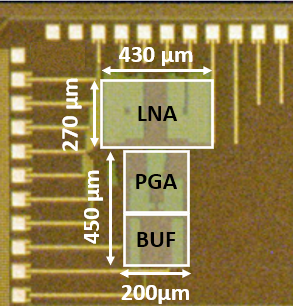##### Fig. 7. Measured AC gain response of AFE IC.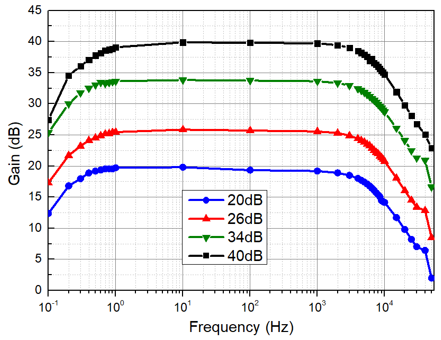##### Fig. 8. Measured input referred noise of AFE IC.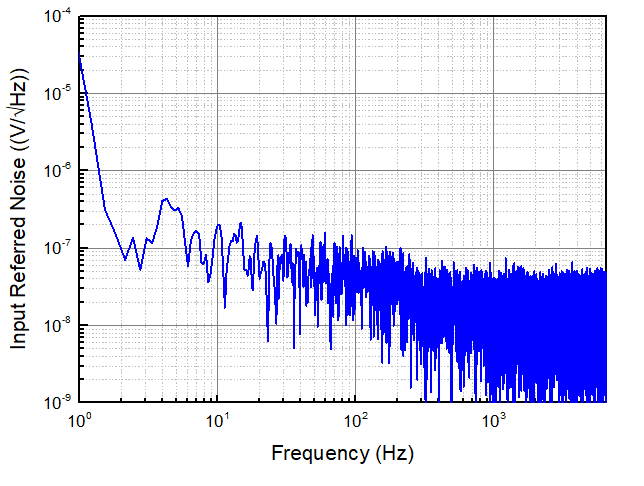##### Fig. 9. Measured AFE output FFT response with artifact.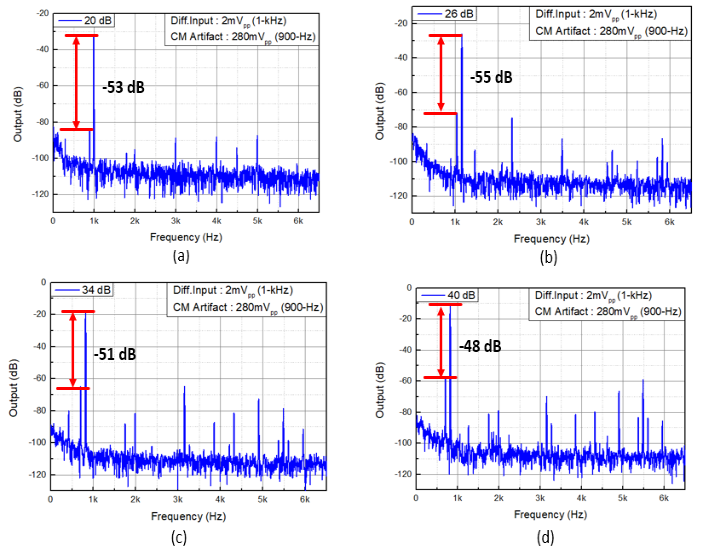##### Fig. 10. Measured AFE output FFT response to 60 mVpp 1-kHz input @ 20 dB gain setting.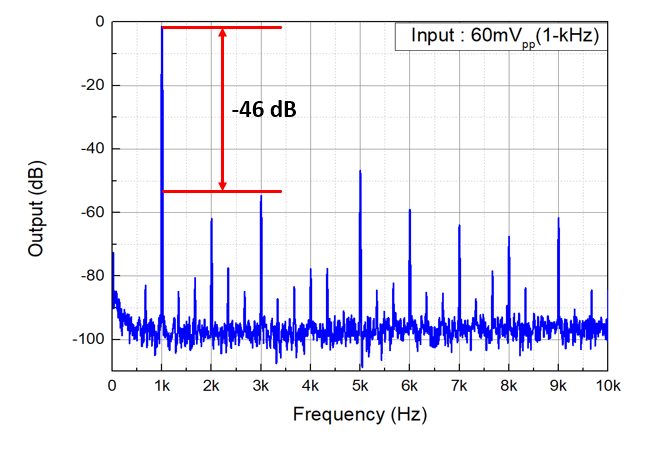##### Fig. 11. Measured CMRR of AFE IC.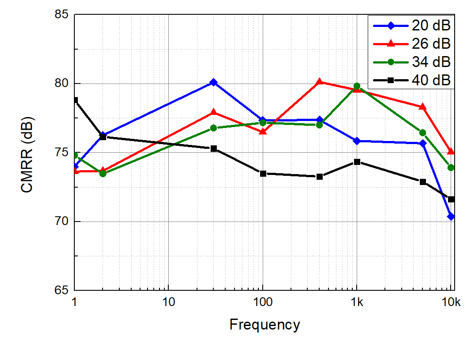##### Fig. 12. A pre-recorded AP signal input using open-source data and waveform generator.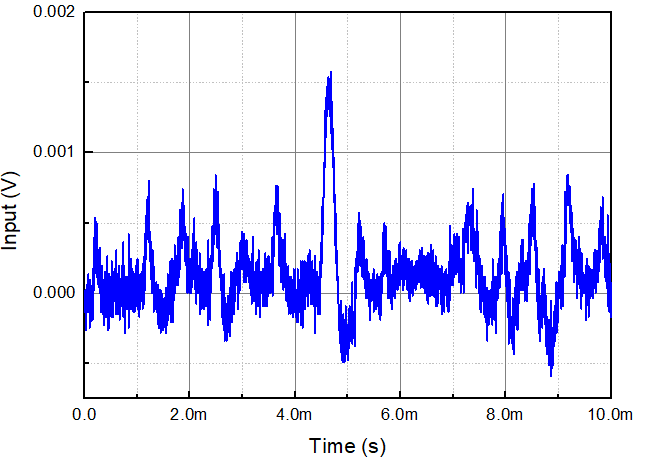##### Fig. 13. Measured pre-recorded AP signal transient response.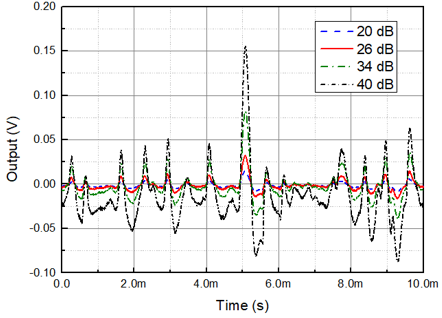##### Fig. 14. Measured AFE output FFT response to the pre-recorded AP signal.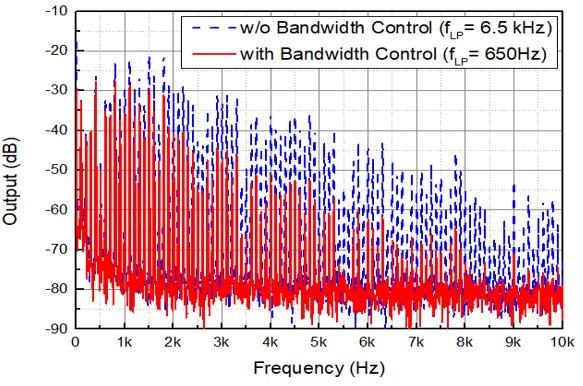## V. CONCLUSIONS

In this paper, a power-efficient analog front-end (AFE) for closed-loop neural interface applications is proposed and implemented using 0.18 ${\mu}$m CMOS process. Comprised of a LNA and a PGA, the mid-band gain of AFE is from 20 to 40-dB. The input-referred noise is measured to be 1.98 ${\mu}$Vrms for 1-650 Hz band and 3.39~${\mu}$Vrms in the 1-6.5 kHz band. For less than 1% of THD, the proposed AFE can handle up to 280 mV$_{\mathrm{pp}}$ of CM artifact and 60 mVpp of DM artifact. The AFE consumes 1.49-${\mu}$W of power from a 1-V supply and occupies an area of 0.21 mm$^{2}$.

## ACKNOWLEDGMENTS

This study was supported by the Research Program funded by Seoultech (Seoul National University of Science and Technology). The EDA tool and MPW fabrication was supported by the IC Design Education Center (IDEC).

## References

1
Liu Y., et al. , Spring 2020, Bidirectional Bioelectronic interfaces, IEEE Solid-State Circuits Mag., pp. 30-462
Harrison R. R., Charles C., Jun. 2003, A low-power low-noise CMOS amplifier for neural recording applications, IEEE J. of Solid-State Circuits, Vol. 38, No. 6, pp. 958-9653
Kim H. S., Cha H.-K., Aug. 2018, An ultra low-power low-noise neural recording analog front-end IC for implantable devices, J. of Semiconductor Technology and Science, Vol. 18, No. 4, pp. 454-4604
Tran L., Cha H.-K., Jan. 2021, An ultra-low-power neural signal acquisition analog front-end IC, Micro-electronics Journal, Vol. 1075
Chandrakumar H., 2017, An 80-mVpp linear-input range, 1.6-G${\Omega}$ Input ompedance, low-power chopper amplifier for closed-loop neural recording that is tolerant to 650-mVpp common-mode interference, IEEE J. of Solid-State Circuits, pp. 1-186
Perez-Prieto N., et al. , May. 2019, Artifact-aware analogue/ mixed-signal front-ends for neural recording applications, in IEEE Intl. Symp. Circuits and Systems (ISCAS)7
Luo D., et al. , Dec. 2020, Design of a low noise bio-potential recorder with high tolerance to power-line interference under 0.8 V power supply, IEEE Trans. on Biomedical Circuits, Systems, Vol. 14, No. 6, pp. 1421-14308
Son J.-Y., Cha H.-K., 2020, An implantable neural stimulator IC with anodic current pulse modulation based active charge balancing, IEEE Access, Vol. 8, pp. 136449-1364589
Denison T., et al. , Dec. 2007, A 2 ${\mu}$W 100 nV/rtHz chopper-stabilized instrumentation amplifier for chronic measurement of neural field potentials, IEEE J. Solid-State Circuits, Vol. 42, No. 12, pp. 2934-294510
Wattanapanitch W., et al. , Jun. 2007, An energy-efficient micropower neural recording amplifier, IEEE Trans. on Biomedical Circuits and Systems, Vol. 1, No. 2, pp. 136-14711
Shen L., Lu N., Sun N., March. 2018, A 1-V 0.25-${\mu}$W inverter stacking amplifier with 1.07 noise efficiency factor, IEEE J. of Solid-State Circuits, Vol. 53, No. 3, pp. 896-90512
J.-C Chung , et al. , May. 2015, An 8-channel power-efficient time-constant-enhanced analog front-end amplifier for neural signal acquisition, in IEEE Intl. Symp. Circuits and Systems (ISCAS)13
Steyaert M. S. J., Sansen W. M. C., Zhongyuan C., Dec. 1987, A micropower low-noise monolithic instrumentation amplifier for medical purposes, IEEE J. Solid-State Circuits, Vol. SC-22, pp. 1163-116814
Lee H.-S., et al. , 2020, A multi-channel neural recording system with adaptive electrode selection for high-density neural interface, in IEEE Engineering in Medicine and Biology Society (EMBC), pp. 4306-430915
Fujisawa S., et al. , 2015, Simultaneous electro-physiological recordings of ensembles of isolated neurons in rat medial prefrontal cortex and intermediate CA1 area of the hippocampus during a working memory task, CRCNS, Vol. 10, pp. K01V5BWK16
Rezaei M., et al. , Apr. 2018, A low-power current-reuse analog front-end for high-density neural recording implants, IEEE Trans. on Biomedical Circuits and Systems, Vol. 12, No. 2, pp. 271-280Donghoon Choi received the B.S. degree in the Department of Mechanical System Design Engi-neering from Seoul National University of Science and Technology (SEOULTECH), Seoul, Korea, in 2020 and the M.S. degree in the Department of Electrical and Information Engineering from SEOULTECH in 2022. In 2022, he joined LX Semicon, Korea, as an analog IC design engineer. His interests include neural interface circuits and ultra-low-power analog circuit design.

Hyouk-Kyu Cha received the B.S. and Ph.D. degrees in electrical engineering at Korea Advanced Institute of Science and Technology (KAIST), Daejeon, Korea, in 2003 and 2009, respectively. From 2009 to 2012, he was a Scientist with the Institute of Microelectronics, (IME), Agency for Science, Technology, and Research (A*STAR), Singapore, where he was involved in the research and development of analog/RF ICs for biomedical applications. Since 2012, he has been with the Department of Electrical and Information Engineering, Seoul National University of Science and Technology, Seoul, Korea, where he is now an Associate Professor. His research interests include low-power CMOS analog/RF IC and system design for biomedical devices.